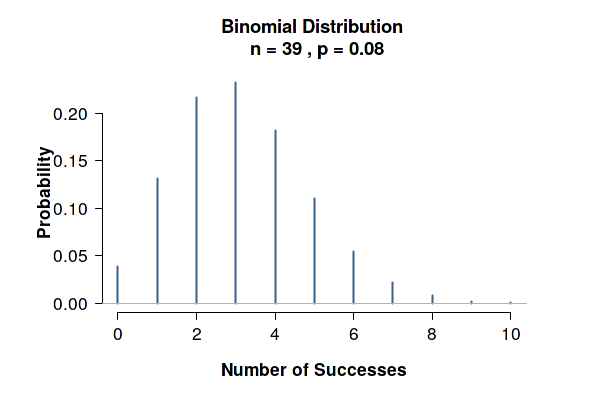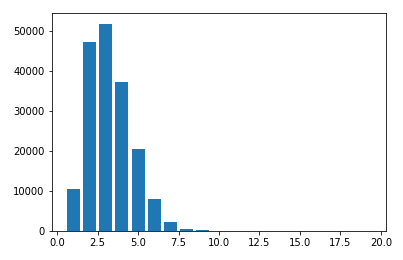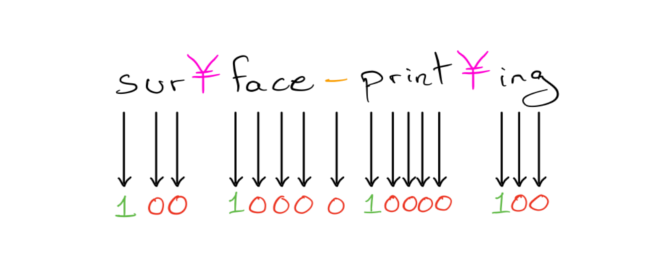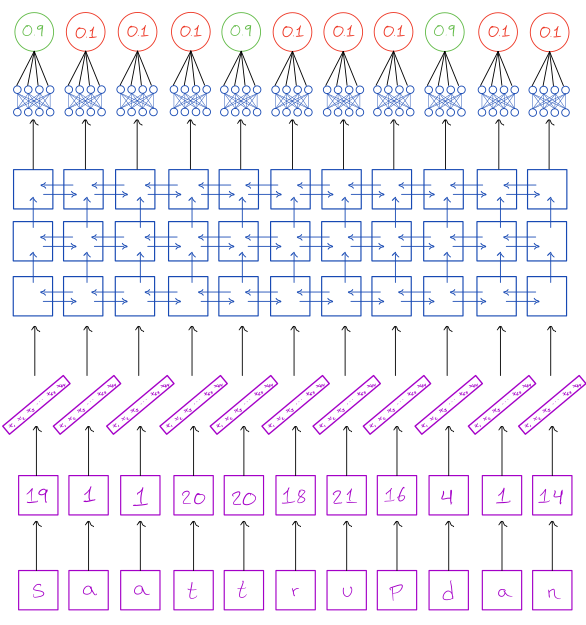As part of another project, I came across the problem of correctly counting the number of syllables in English words. After searching around and seeing mostly rule- and dictionary-based methods, I ended up building such a syllable counter from scratch, which ultimately led to the construction of a neural network model that achieved a 96.89% validation accuracy on this task. Here I’ll go through the journey that ended up with that final product.

All code can be found in the github repo and all the data, including the model itself, can be found in the pcloud repo.

## Getting the data

The first question, as always, is getting hold of a dataset. I considered both the CMU phoneme dataset and the Gutenberg Moby Hyphenator II dataset, the former containing the phonemes for ~130k English words and the latter containing the hyphenation of ~170k English words. Even though phonemes might make it easier for a model to find the correct number of syllables I opted for the larger Gutenberg dataset. Quantity over quality.

The Gutenberg corpus consists of English words in which the hyphenation points are marked with the Yen symbol (¥) if you decode the file using the latin-1 encoding. Several lines have multiple words, separated by spaces.

a cap¥pel¥la\n
well-rat¥ed\n
un¥pur¥chas¥a¥ble\n
Twee¥dle¥dum and Twee¥dle¥dee

One thing that I found amusing is that the number of syllables in the corpus roughly follows a binomial distribution:## Preprocessing

### Target values

The first task was then to convert these strings to a dataset more appropriate for my needs. I started by pulling out my vocabulary by separating the lines by spaces, and by summing up all the non-letter symbols (and add 1) I could get the syllable counts. So far so good! My naive first attempt was then to use the (cleaned) words and syllable counts and simply interpret the task as a regression task that can be optimised with the mean squared loss. This did not work well, which can be due to a couple of different factors:

• Firstly, this is an ordinal regression task, i.e. my outcomes should be integers, and thus my bog standard regression tools ostensibly don’t work well here. A way to combat this is to treat the task as a classification task, where class $n$ is true if and only if the outcome has at least $n$ many syllables. A downside to this approach is that we have to then assign an upper bound to the number of syllables in a word, which my mathematical mind really did not like the sound of.
• Secondly, and perhaps more importantly, I did not take into account all the information in the dataset! Indeed, it provides information about not just how many syllables a word has, but also where they occur.

These points made me change the task from a regression problem to a sequence-to-sequence task, in which we convert a sequence of characters to a sequence of probabilities of the same length, where the probability indicates how likely it is that the given character starts a new syllable. Since the input- and output sequences have the same length we don’t have to deal with an encoder-decoder framework and can simply use a recurrent cell as-is.### Words

With the preprocessing of the target labels complete, the remaining part of the preprocessing is standard: we need to split the words into sequences of characters, convert the characters to integers and then pad these sequences such that all sequences in a given batch have the same length. This can be done quite easily with the torchtext library:

def get_data(file_name, batch_size = 32, split_ratio = 0.99):
from torchtext.data import Field, TabularDataset, BucketIterator

# Define fields in dataset, which also describes how the fields should
# be processed and tokenised
TXT = Field(tokenize = lambda x: list(x), lower = True)
SEQ = Field(tokenize = lambda x: list(map(int, x)), pad_token = 0,
unk_token = 0, is_target = True, dtype = torch.float)
NUM = Field(sequential = False, use_vocab = False,
preprocessing = lambda x: int(x), dtype = torch.float)

datafields = [('word', TXT), ('syl_seq', SEQ), ('syls', NUM)]

# Load in dataset, applying the preprocessing and tokenisation as
# described in the fields
dataset = TabularDataset(
path = os.path.join('data', f'{file_name}.tsv'),
format = 'tsv',
fields = datafields
)

# Split dataset into a training set and a validation set in a
# stratified fashion, so that both datasets will have the same
# syllable distribution.
train, val = dataset.split(
split_ratio = split_ratio,
stratified = True,
strata_field = 'syls'
)

# Build vocabularies
TXT.build_vocab(train)
SEQ.build_vocab(train)

# Split the two datasets into batches. This converts the tokenised
# words into integer sequences, and also pads every batch so that,
# within a batch, all sequences are of the same length
train_iter, val_iter = BucketIterator.splits(
datasets = (train, val),
batch_sizes = (batch_size, batch_size),
sort_key = lambda x: len(x.word),
sort_within_batch = False,
)

# Apply custom batch wrapper, which outputs the data in the form
# that the network wants it
train_dl = BatchWrapper(train_iter)
val_dl = BatchWrapper(val_iter)

return train_dl, val_dl


Notable here is the BucketIterator object, which sorts the dataset by the length of the words and then puts words of similar lengths into the same batches, to minimise the padding needed per batch. Therefore we don’t need to worry about padding every character sequence to the length of the longest word.

The BatchWrapper class at the end of the function above is a very simple class that outputs only the word and the binary syllable sequence. The reason why I even included the syls field to start with was because I use it when I’m splitting the dataset into training- and validation sets above, as I’m splitting it in a stratified fashion. This will result in a validation set with the same binomial-like syllable distribution as we saw above.

class BatchWrapper:
''' A wrapper around a dataloader to pull out data
in a custom format. '''

def __init__(self, dl):
self.dl = dl
self.batch_size = dl.batch_size

def __iter__(self):
for batch in self.dl:
yield (batch.word, batch.syl_seq)

def __len__(self):
return len(self.dl)


## Building a model

Now, with the preprocessing all done, it’s time to build a model! As we’re mapping a sequence of length $n$ to a sequence of length $n$, a simple recurrent neural network should be able to do that job. I was playing around with attention mechanisms as well, both as an addition to the network as well as a replacement for the recurrent cells, but both resulted in poorer performance. This might just be due to these mechanisms being mostly suited for encoder-decoder frameworks, in which the lengths of the input sequence and output sequence differ.

After trying out a bunch of different things, here is the (quite simple!) architecture that turned out to work the best for me:Concretely, this converts the characters into 64-dimensional vectors, processes them in three bi-directional GRU layers with 2x128 = 256 hidden units, followed by a time-distributed fully connected layer with 256 units, and finally every 256-dimensional vector is projected down to a single dimension, yielding our logits, to which we apply a sigmoid function at every timestep.

This model has 810,369 trainable parameters, which doesn’t seem completely out of proportions as we’re dealing with ~170k words in our training set, each of which has ~4 characters on average, amounting to ~680k character inputs.

As for regularisation I went with the following:

• Dropout between every hidden layer, with a dropout rate of 20% between the recurrent layers and 50% before the dense layer
• Weighted labels, where I gave a higher weight to the positive instances, since the number of 0’s and 1’s are not equally distributed
• A very small batch size, which enforces more randomness into the weights and thereby increasing the variance. I went with batches of size 8

In terms of loss functions I could just use binary cross entropy, but the problem with that is that then I won’t really be evaluating the entire word but only the individual characters locally. As we’re ultimately interested in a syllable count we need to ensure that the output numbers depend on each other. After testing a few different ones, I ended up choosing the average of the binary cross entropy and the root mean squared error:

def bce_rmse(pred, target, pos_weight = 1.3, epsilon = 1e-12):
''' A combination of binary crossentropy and root mean squared error.

INPUT
pred
A 1-dimensional tensor containing predicted values
target
A 1-dimensional tensor containing true values
pos_weight = 1.3
The weight that should be given to positive examples
epsilon = 1e-12
A small constant to avoid dividing by zero

OUTPUT
The average of the character-wise binary crossentropy and the
word-wise root mean squared error
'''

# Weighted binary cross entropy
loss_pos = target * torch.log(pred + epsilon)
loss_neg = (1 - target) * torch.log(1 - pred + epsilon)
bce = torch.mean(torch.neg(pos_weight * loss_pos + loss_neg))

# Root mean squared error
mse = (torch.sum(pred, dim = 0) - torch.sum(target, dim = 0)) ** 2
rmse = torch.mean(torch.sqrt(mse + epsilon))

return (bce + rmse) / 2


## Converting the output to syllables

Given an output sequence $\langle x_1, \dots, x_n \rangle\in (0,1)^n$ of the model, how do we convert this into a syllable count?

We could firstly round the probabities to either 0 or 1 and then simply sum them up. This turned out to not be ideal however, because in the above loss function we’re taking the root mean squared error of the probabilities and not the rounded values (this wouldn’t be differentiable), which means that the model will be doing its best to ensure that the sum of the probabilities will equal the syllable count. So, we will therefore follow the loss function and sum the probabilities.

## Results

After tuning the hyperparameters, the best model achieved a 96.89% validation accuracy and a 97.53% training accuracy.

Here are some of the words that the model counted wrongly:

Word Syllables Model prediction
siobhan 2 3
xtacihuatl 4 5
init 1 2
bandaranaike 6 4
lemonnier 3 4
molise 3 2
inertia 3 4
liwn 2 1
siena 3 2
parnaiba 4 3
aphrodite 4 3
glacing 3 2